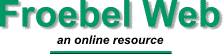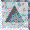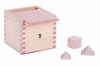This gift expands on the cubes of the third gift. Presented as a larger cube with three blocks along each edge, it would theoretically consist of twenty seven cubes. The surprise in this gift is that three of the cubes are divided diagonally to form six triangular faced blocks and another three are divided twice to form twelve smaller triangular blocks. A 3" cube therefore divides into 39 blocks; 21 one-inch cubes, 6 triangle half cubes, and 12 triangle quarter cubes.

Four of the larger triangle half cubes can be arranged to form a larger square. The same size square can also be formed by arranging four of the smaller triangle quarter cubes around a cube. This provides the basis for an elegant demonstration of Pythagorus' Theorum, that the square on the hypotemuse is equal to the sum of the squares on the other two sides.

The triangular shapes also enable the construction of more complex Beauty and Life Forms.

This concludes the original five gifts that Froebel published in his life time. The remaining gifts were in used by Froebel in his Kindergarten and published after his death. The sixth gift is a useful extension of the blocks. The remaining gifts extend the exploration of solids to surfaces and lines, Thus moving from the concrete to the abstract representation of solids using lines.

understanding block play